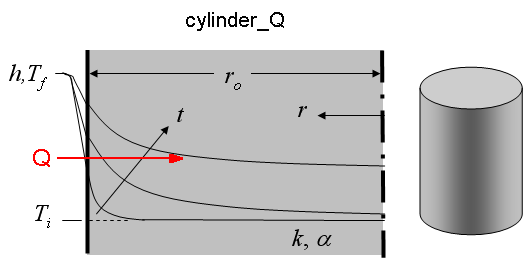Contents - Index

Cylinder_QFunction cylinder_Q(time, T_i, T_f, alpha, k, h, r_o) returns the heat transfer per unit length [J/m or Btu/ft] for a long right circular cylinder with the exterior walls (r=r_o) exposed to convection.

Inputs:
time - time relative to beginning of convection [sec]
T_i - initial temperature of cylinder [C], [K], [F], or [R]
T_f - bulk fluid temperature [C], [K], [F], or [R]
alpha - thermal diffusivity [m^2/s] or [ft^2/hr]
k - thermal conductivity of cylinder [W/m-K] or [Btu/hr-ft-R]
h - heat transfer coefficient [W/m^2-K] or [Btu/hr-ft^2-R]
r_o - outer radius of cylinder [m] or [ft]

Notes:
Function cylinder_Q determines non-dimensional Biot and Fourier numbers and then calls the function cylinder_Q_ND, which determines the non-dimensional heat transfer, Q_hat, as explained in section 3.5.2 of Nellis and Klein.

Example:
\$UnitSystem SI K Pa J
time=3000 [sec]
T_i=303 [K]
T_f=363 [K]
alpha=8.608e-5 [m^2/s]
k= 233 [W/m-K]
h= 45 [W/m^2-K]
r_o= 0.1 [m]
Q=cylinder_Q(time, T_i, T_f, alpha, k, h, r_o)

{Solution: Q=3.213E+06 [J/m]}

Transient Conduction Index